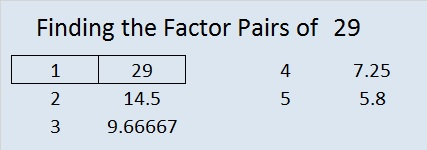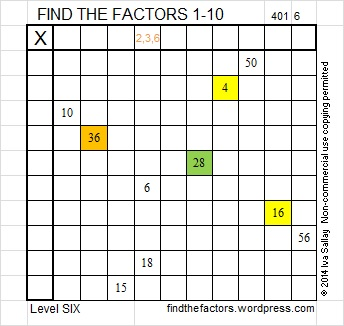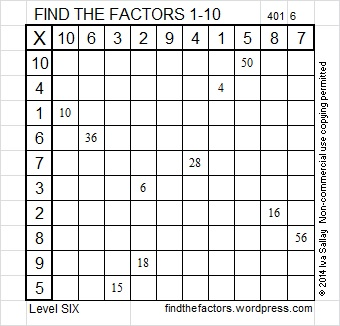# 29 and A Level 6 Snowball

• 29 is a prime number.
• Prime factorization: 29 is prime.
• The exponent of prime number 29 is 1. Adding 1 to that exponent we get (1 + 1) = 2. Therefore 29 has exactly 2 factors.
• Factors of 29: 1, 29
• Factor pairs: 29 = 1 x 29
• 29 has no square factors that allow its square root to be simplified. √29 ≈ 5.38516How do we know that 29 is a prime number? If 29 were not a prime number, then it would be divisible by at least one prime number less than or equal to √29 ≈ 5.4. Since 29 cannot be divided evenly by 2, 3, or 5, we know that 29 is a prime number.

29 is never a clue in the FIND THE FACTORS puzzles.

Here is a FIND THE FACTORS 1-12 puzzle that is shaped like a snowball. Because it is a level 6 puzzle, you might feel as if you’re caught in a snowball fight:The object of the puzzle is to write the numbers 1 to 12 in the top row and again in the first column so that those numbers are the factors of the given clues. If you have attempted a level 6 puzzle, you may have screamed, “IS IT EVEN POSSIBLE to solve a level 6 puzzle without guessing and checking?” I promise you it is, BUT you will need to look at ALL the clues in the puzzle and think about them logically. I don’t want to spoil any of the fun of this week’s puzzle, so I will explain in detail how to solve last week’s puzzle instead.

Notice that for last week’s puzzle there is only one row or column with more than one clue, the column with the 6 and 18 in it. Notice there are three possible common factors, 2, 3, and 6 that will allow us to write only the numbers from 1 to 10 in the factor row and the factor column. You may be tempted to guess and check, but doing that often leads to frustration and/or screaming, so let’s ignore that column for now.As we examine the other clues, we notice that there are four clues that are divisible by four: 4, 16, 28, and 36. Let’s look at the possible factors for each of those clues.Remember that each number from 1 to 10 can only appear once in the factor row and once in the factor column. That means that if 4 is 2 x 2, then 16 must be 4 x 4. Likewise if 16 is 2 x 8, then 4 must be 1 x 4.

If you think about it, you will realize that for this puzzle 4 MUST be a factor of either 4 OR 16. You should also notice that 4 must be a factor of 28. Therefore, in this puzzle, 4 will NOT be one the factors of 36.

We therefore, know that 6 x 6 = 36 works for this puzzle. Since that uses up both 6s, we know that we can’t use 6 as one of the factors of 18, and since 6 x 3 = 18, we also can’t use 3 as its factor. Logic tells us that 2 is the only common factor of 6 and 18 that will work for this puzzle. We now can fill in the corresponding factor cells from the information we have learned so far:Since we found a 9 in the factor column, try to figure out where the 9 goes in the factor row. Since none of the clues that are left are divisible by 9, there is only one place a 9 can go in the factor row: over the column with no clues.

Now if we complete the puzzle using the clues in the order listed on the chart below, we can feel the rhythm as we fill in the rest of the factor cells. The first factor of each multiplication product should be written in the factor row (and the second factor should be written in the factor column):Now we have found all the factors using LOGIC only. We did not guess and check at all. Last week’s completed puzzle is shown below.Try to discover the secret of the snowball puzzle on your own as well. This week’s puzzles are also available in an excel file here, if you have a spreadsheet program on your computer you can access it. If you enable editing in excel, you can type your answers directly onto the puzzle, and you can also easily print the puzzles. Good luck!

This site uses Akismet to reduce spam. Learn how your comment data is processed.